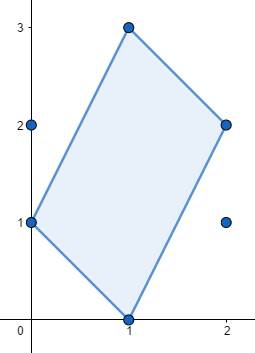Maximum Polygon
Tag(s):

Algorithms, Convex Hull, Geometry, Hard, Searching algorithm, Ternary search

Problem
Editorial
Analytics

Given $N$ points on plane, the $N$ points forms a convex polygon. You need to find $M$ points among them, then we calculate the convex hull of the $M$ points.

You need to maximize $\frac{The\ area\ of\ the\ convex\ hull}{The\ perimeter\ of\ the\ convex\ hull}$.

Input Format

First line contains two integers $N,M(2\le M\le N\le 120)$.

Each of the next $N$ lines contains two integers $X_i,Y_i(-10^9\le X_i,Y_i\le 10^9)$, represent a point.

It's guaranteed no two points coincide.

Output Format

One float number, represent the answer, round to 4 decimal digits. It's guaranteed the output will not change if we add $10^{-6}$ to the answer or subtract $10^{-6}$ from the answer.

Note : The statement for this problem has been updated. We are rejudging all submissions, please check announcement on contest page for detailed issue.

SAMPLE INPUT
6 4
1 0
2 1
2 2
1 3
0 1
0 2

SAMPLE OUTPUT
0.4109

ExplanationThe sample is in the above graph. $Area=3,Perimeter\approx 7.300563$.

Time Limit: 5.0 sec(s) for each input file.
Memory Limit: 256 MB
Source Limit: 1024 KB
Marking Scheme: Marks are awarded when all the testcases pass.
Allowed Languages: Bash, C, C++, C++14, Clojure, C#, D, Erlang, F#, Go, Groovy, Haskell, Java, Java 8, JavaScript(Rhino), JavaScript(Node.js), Julia, Kotlin, Lisp, Lisp (SBCL), Lua, Objective-C, OCaml, Octave, Pascal, Perl, PHP, Python, Python 3, R(RScript), Racket, Ruby, Rust, Scala, Swift, Swift-4.1, TypeScript, Visual Basic

CODE EDITOR

Initializing Code Editor...

ContributorChallenge Name

September Circuits '18

OTHER PROBLEMS OF THIS CHALLENGE
• Basic Programming > Implementation
• Algorithms > Sorting
• Basic Programming > Recursion
• Algorithms > Dynamic Programming
• Data Structures > Trees